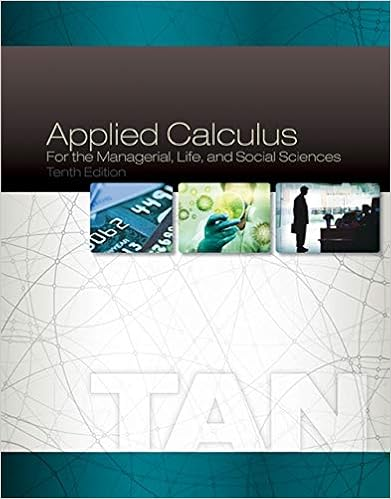# Pts; Review from MFE 1 Let f x, y = x 2 + 2 xy 2 +...

• Homework Help
• 1
• 58% (12) 7 out of 12 people found this document helpful

This preview shows page 1 out of 1 page.

##### We have textbook solutions for you!
The document you are viewing contains questions related to this textbook.The document you are viewing contains questions related to this textbook.
Chapter 8 / Exercise 4
Applied Calculus for the Managerial, Life, and Social Sciences
TanExpert Verified
Math for Econ II, Written Assignment 3 (28 points) Due Friday, February 21st New York University Please write neat solutions for the problems below. Show all your work. If you only write the answer with no work, you will not be given any credit. Write your name and recitation section number. Staple your homework if you have multiple pages! 1. (4 pts; Review from MFE 1) Let f ( x, y ) = x 2 + 2 xy 2 + 2 y 2 . Find all critical points of f , and classify each critical point as a local min, local max, or saddle point. Does f have a global maximum value? Briefly justify. 2. (4 pts) Use Lagrange multipliers to find the maximum and minimum values of f subject to the given constraint if such values exist. If there is no minimum, justify why. If there is no maximum, justify why. f ( x, y ) = x 2 + y , x 2 - y 2 = 1 3. (7 points) Use Lagrange multipliers to find the maximum and minimum values of f subject to the given constraint if such values exist. If there is no minimum, justify why. If there is no maximum, justify why. f ( x, y ) = x 2 y + 3 y 2 - y, x 2 + y 2 10 .
##### We have textbook solutions for you!
The document you are viewing contains questions related to this textbook.The document you are viewing contains questions related to this textbook.
Chapter 8 / Exercise 4
Applied Calculus for the Managerial, Life, and Social Sciences
TanExpert Verified
•••